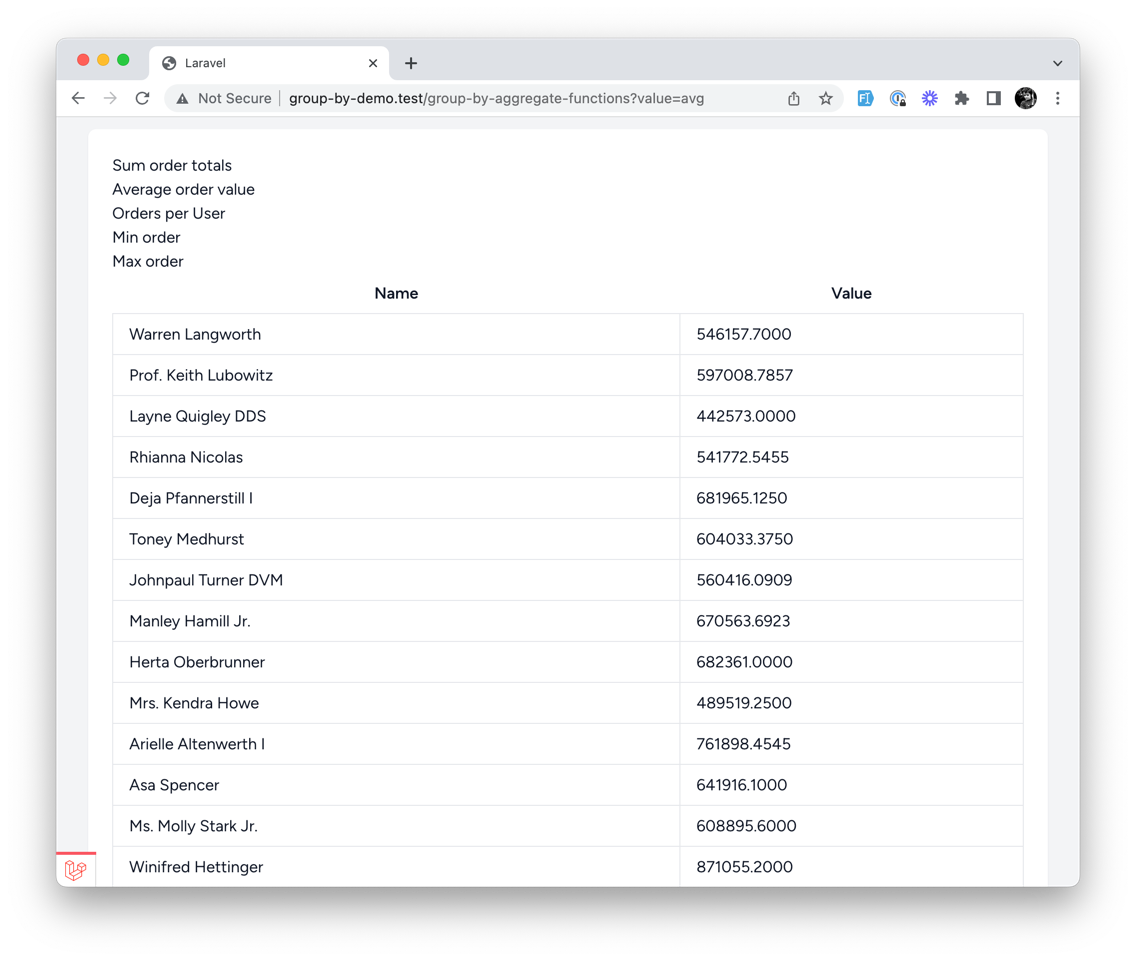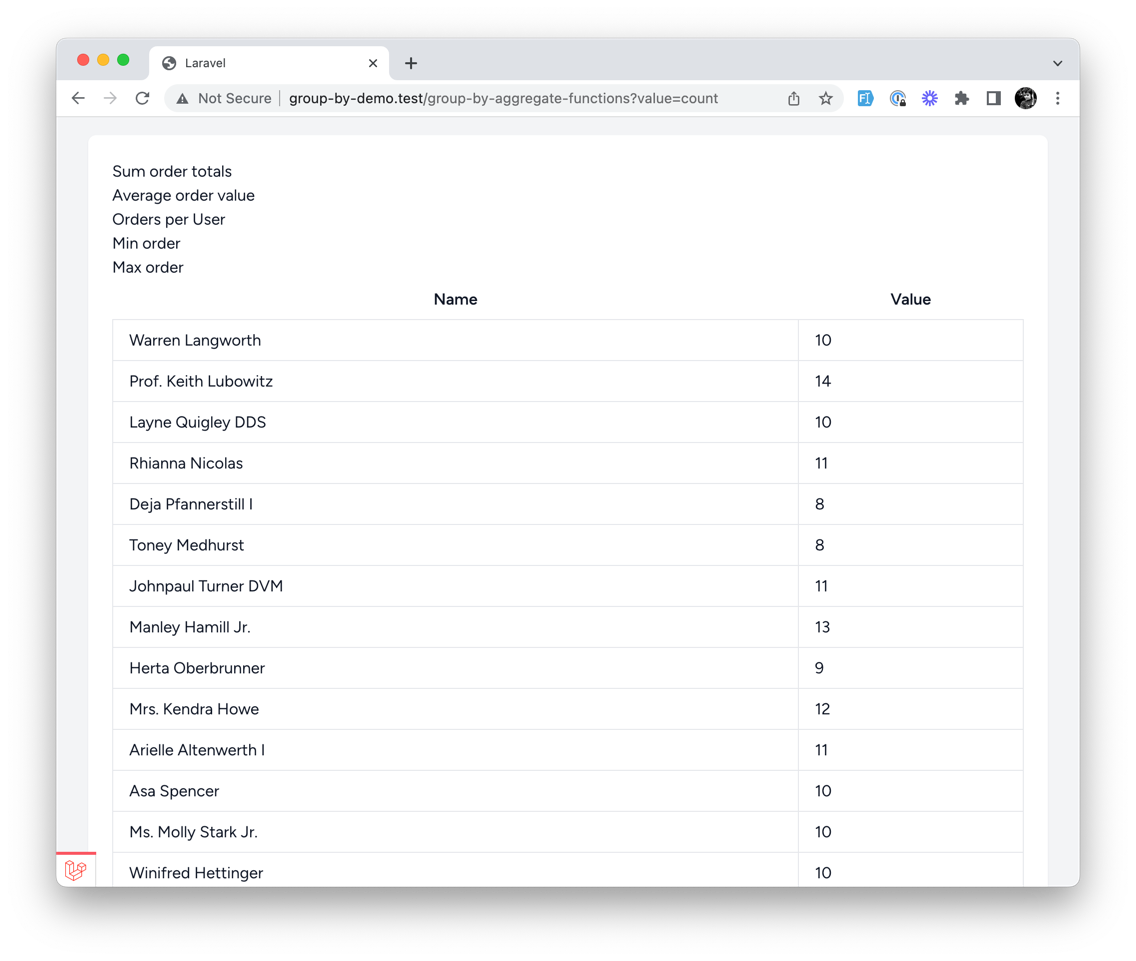Courses

# Example 2. GROUP BY Aggregation: SUM, AVG, MIN, MAX, COUNT

This example will show you how to group by a column and aggregate data from other columns. For example, we need to display the average order total for each user:In this case, let's make an all-in-one example for all those functions.

Controller

``use App\Models\Order;use Illuminate\Http\Request;use Illuminate\Support\Facades\DB; // ... \$orders = Order::query(); switch (\$request->input('value')) {    case 'sum':    default:        \$orders = \$orders->select('user_id', DB::raw('sum(total) as value'));        break;    case 'avg':        \$orders = \$orders->select('user_id', DB::raw('avg(total) as value'));        break;    case 'min':        \$orders = \$orders->select('user_id', DB::raw('min(total) as value'));        break;    case 'max':        \$orders = \$orders->select('user_id', DB::raw('max(total) as value'));        break;    case 'count':        \$orders = \$orders->select('user_id', DB::raw('count(total) as value'));        break;} \$orders = \$orders->with('user:name,id')    ->groupBy('user_id')    ->get(); return view('examples.groupByAggregateFunctions', [    'orders' => \$orders]);``

As you can see, we have a variety of functions we use to display different types of data:

• `sum()` - Display total order values for each user
• `avg()` - Display average order values for each user
• `min()` - Display minimum order values for each user
• `max()` - Display maximum order values for each user
• `count()` - Display the total number of orders for each user

All of this lands in the `value` column from this SQL query:

``select `user_id`, sum(total) as valuefrom `orders`group by `user_id`# orselect `user_id`, avg(total) as valuefrom `orders`group by `user_id`# orselect `user_id`, min(total) as valuefrom `orders`group by `user_id`# orselect `user_id`, max(total) as valuefrom `orders`group by `user_id`# orselect `user_id`, count(total) as valuefrom `orders`group by `user_id```

Which we can display in our view along with a simple navigation to switch between the different functions:

View

``{{-- ... --}}<ul>    <li>        <a href="{{ route('group-by-aggregate-functions', ['value' => 'sum']) }}">            Sum order totals        </a>    </li>    <li>        <a href="{{ route('group-by-aggregate-functions', ['value' => 'avg']) }}">            Average order value        </a>    </li>    <li>        <a href="{{ route('group-by-aggregate-functions', ['value' => 'count']) }}">            Orders per User        </a>    </li>    <li>        <a href="{{ route('group-by-aggregate-functions', ['value' => 'min']) }}">            Min order        </a>    </li>    <li>        <a href="{{ route('group-by-aggregate-functions', ['value' => 'max']) }}">            Max order        </a>    </li></ul> <table class="table-auto w-full">    <thead>    <tr>        <th class="px-4 py-2">Name</th>        <th class="px-4 py-2">Value</th>    </tr>    </thead>    <tbody>    @foreach (\$orders as \$order)        <tr>            <td class="border px-4 py-2">{{ \$order->user->name }}</td>            <td class="border px-4 py-2">{{ \$order->value }}</td>        </tr>    @endforeach    </tbody></table>{{-- ... --}}``

In the end, we have a simple navigation that allows us to switch between different aggregate functions and display the data in a table, like total order count by user:Here's a nice modern php match statement folks can use instead of switch case from the controller above:

``````        \$orders = Order::query();

\$value = \$request->input('value');

\$orders = match (\$value) {
'avg' => \$orders->select('user_id', DB::raw('avg(total) as value')),
'min' => \$orders->select('user_id', DB::raw('min(total) as value')),
'max' => \$orders->select('user_id', DB::raw('max(total) as value')),
'count' => \$orders->select('user_id', DB::raw('count(total) as value')),
default => \$orders->select('user_id', DB::raw('sum(total) as value')),
};
``````
👍 3